Function Repository Resource:

# FindEquationalPath

Find a path that goes from one expression to another with a sequence of replacements

Contributed by: Nikolay Murzin
 ResourceFunction["FindEquationalPath"][theorem, axioms] finds a replacement path from the left-hand side of a theorem to its right-hand side using replacements specified by axioms. ResourceFunction["FindEquationalPath"][proof] find a path for the precomputed ProofObject proof. ResourceFunction["FindEquationalPath"][…, hypothesis] specify an explicit hypothesis lemma from the underlying ProofObject to return a path for. ResourceFunction["FindEquationalPath"][…,hypothesis,conclusion] specify an explicit conclusion lemma from the underlying ProofObject to be used in path construction. ResourceFunction["FindEquationalPath"][…,prop] returns a specified property prop of a replacement path.

## Details and Options

ResourceFunction["FindEquationalPath"] uses FindEquationalProof to find a proof of equational proposition and by using cut-elimination, derives a sequential path of one-step replacements from one side of a theorem to another.
The replacement path consists of pattern expressions corresponding to logic formulas with patterns (like a Blank, a_) representing universal variables (ForAll) and other symbols representing functional constants (Exists).
Properties prop supported by ResourceFunction["FindEquationalPath"] include:
 "ProofObject" the corresponding ProofObject "Path" a list of expressions comprising a found path (default) "Justification" a list of axioms used in each subsequent step of a path together with its orientation and position "Rewrites" a list of rewriting functions that reproduce a path "RewritesTest" test rewriting functions to successfully reproduce a path All all of the above as an association
For the "Justification" property, axiom orientation is a direction of a corresponding rule, for example a==b can be used as a b (right orientation) or b a (left orientation).
Position of a step is a position of a subexpression at which a rule corresponding to an axiom is being applied.
Explicit hypothesis and conclusion lemmas are keys from the "Proof" or "ProofDataset" properties of a ProofObject, with {"Hypothesis", 1} and corresponding {"Conclusion", 1} being the default.
Explicit conclusion lemma is rarely needed, it is used as a starting point to generate a path by backtracking through a proof graph.
ResourceFunction["FindEquationalPath"] takes the option "Reverse". With "Reverse"True, a reversed replacement path is returned.

## Examples

### Basic Examples (3)

Find a replacement path given a set of equations:

 In:=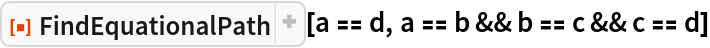Out=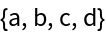Find a replacement path using universally quantified equations:

 In:=Out=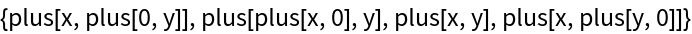Find a much more complicated path:

 In:=Out=### Scope (7)

Use names from the AxiomaticTheory for a list of axioms and its notable theorems:

 In:=Out=Specify a custom theorem with AxiomaticTheory axioms:

 In:=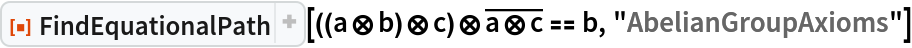Out=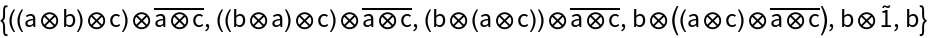Use a ProofObject to find a replacement path:

 In:=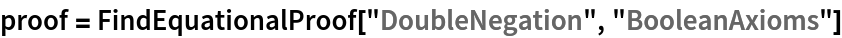Out=In:=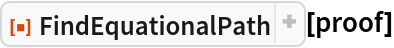Out=Specify a lemma or a different hypothesis from a proof to construct a path for:

 In:=Out=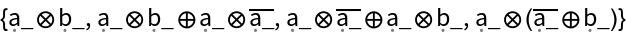In:=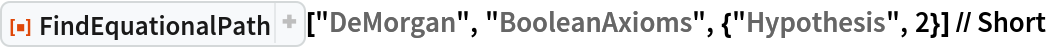Out=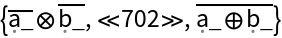Find a replacement path in a string substitution system:

 In:=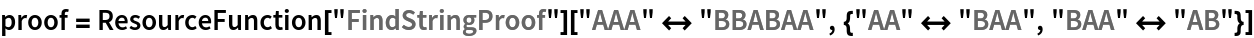Out=In:=Out=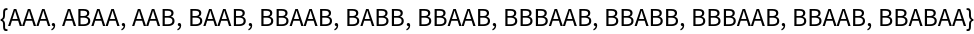Find a replacement path in a Wolfram model system:

 In:=Out=In:=Out=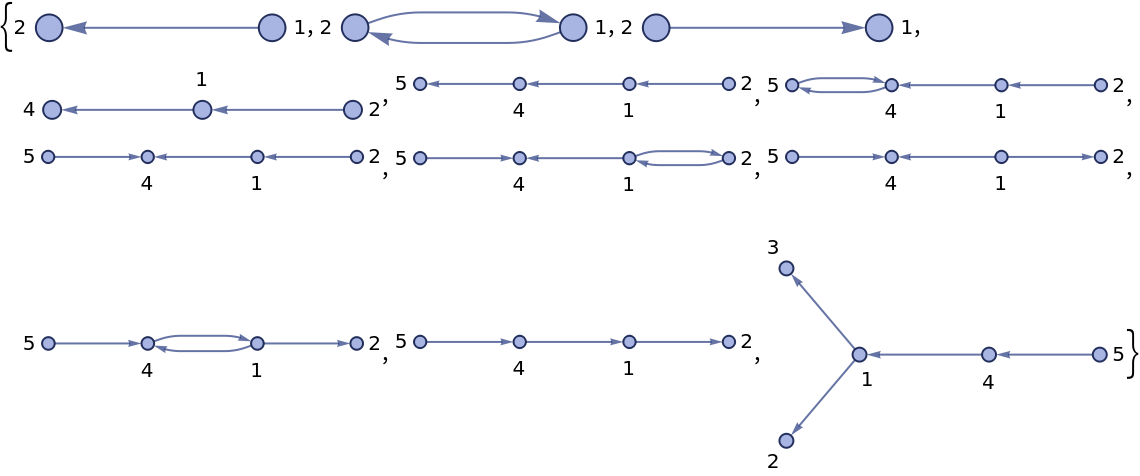Reproduce the replacement path using rewriting functions:

 In:=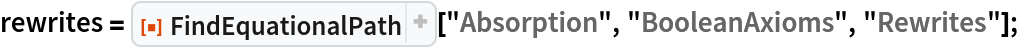In:=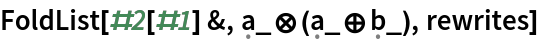Out=### Options (1)

#### Reverse (1)

Reverse a path:

 In:=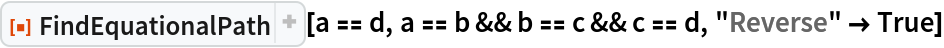Out=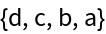### Neat Examples (3)

Find a replacement path for a well known syllogism by specifying an existentially quantified goal:

 In:=Out=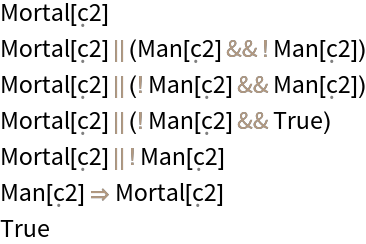Test Boolean theorems:

 In:=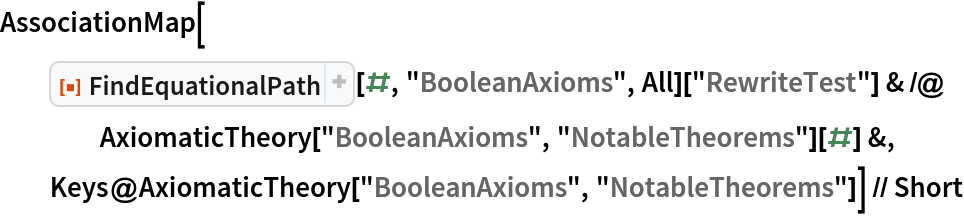Out=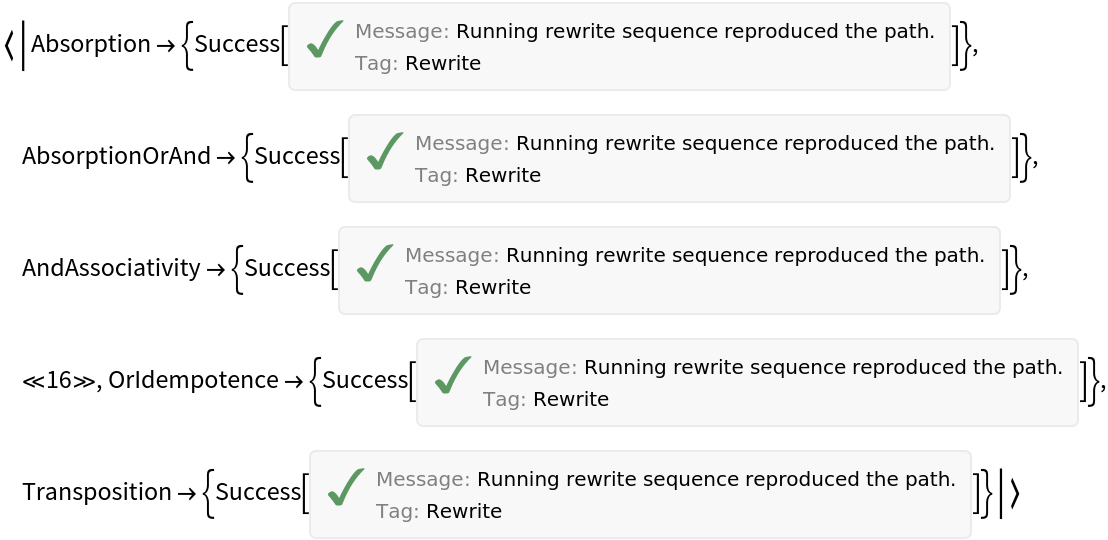An example from A New Kind of Science (p. 775):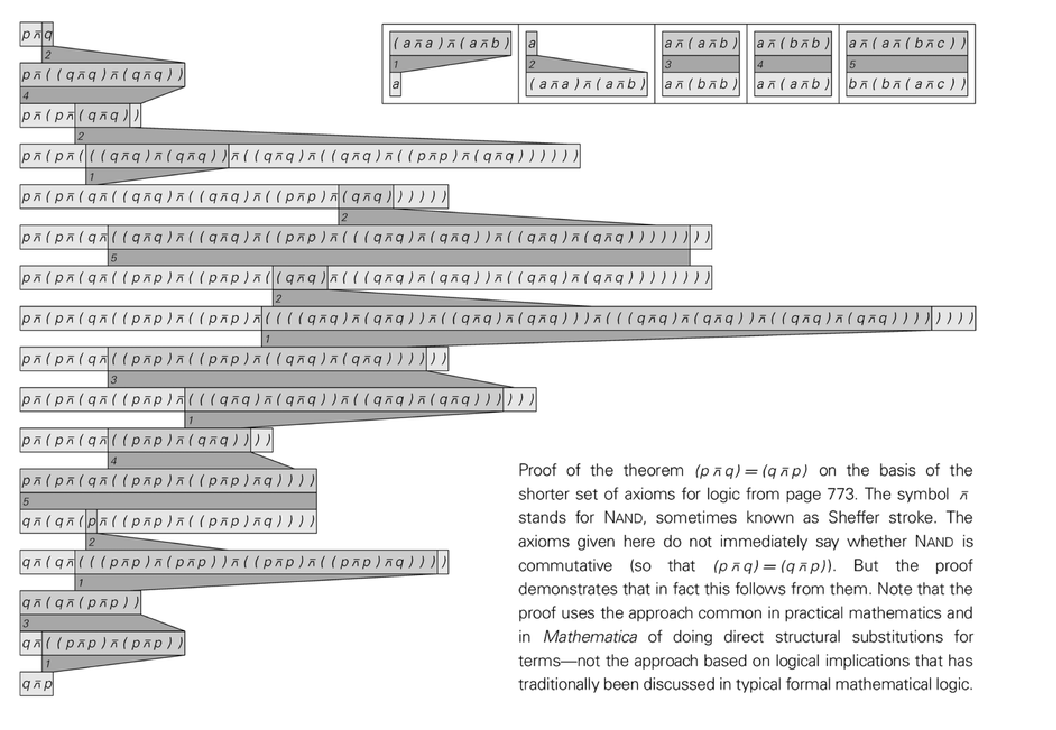In:=Out=In:=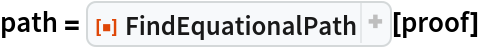Out=It is two steps shorter, but it uses a reverse of one axiom:

 In:=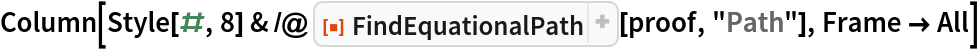Out=Prove the existential version of the same theorem using a proof by refutation:

 In:=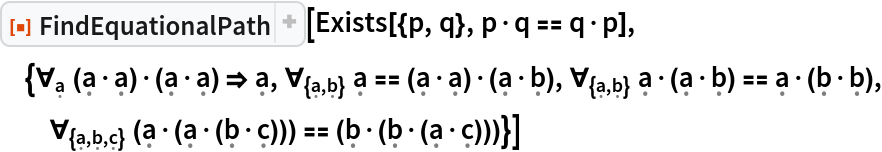Out=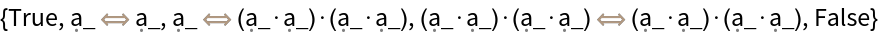## Version History

• 1.0.1 – 25 January 2023
• 1.0.0 – 20 January 2023